Odds and Odds Ratio Estimation

In models with a logit, generalized logit, or cumulative logit link, you can obtain estimates of odds ratios through the ODDSRATIO options in the PROC GLIMMIX, LSMEANS, and MODEL statements. This section provides details about the computation and interpretation of the computed quantities. Note that for these link functions the EXP option in the ESTIMATE and LSMESTIMATE statements also produces odds or odds ratios.

Consider first a model with a dichotomous outcome variable, linear predictor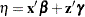, and logit link function. Suppose that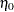represents the linear predictor for a condition of interest. For example, in a simple logistic regression model with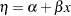,might correspond to the linear predictor at a particular value of the covariate—say,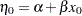.

The modeled probability is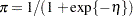, and the odds for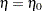are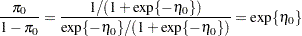Becauseis a logit, it represents the log odds. The odds ratio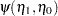is defined as the ratio of odds for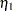and,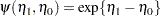The odds ratio compares the odds of the outcome under the condition expressed byto the odds under the condition expressed by. In the preceding simple logistic regression example, this ratio equals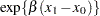. The exponentiation of the estimate ofis thus an estimate of the odds ratio comparing conditions for which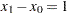. Ifand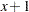represent standard and experimental conditions, for example,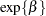compares the odds of the outcome under the experimental condition to the odds under the standard condition. For many other types of models, odds ratios can be expressed as simple functions of parameter estimates. For example, suppose you are fitting a logistic model with a single classification effect with three levels:

proc glimmix;
class A;
model y = A / dist=binary;
run;

The estimated linear predictor for levelof A is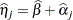,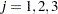. Because thematrix is singular in this model due to the presence of an overall intercept, the solution for the intercept estimates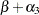, and the solution for theth treatment effect estimates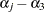. Exponentiating the solutions for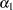and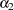thus produces odds ratios comparing the odds for these levels against the third level of A.

Results designated as odds or odds ratios in the GLIMMIX procedure might reduce to simple exponentiations of solutions in the "Parameter Estimates" table, but they are computed by a different mechanism if the model contains classification variables. The computations rely on general estimable functions; for the MODEL, LSMEANS, and LSMESTIMATE statements, these functions are based on least squares means. This enables you to obtain odds ratio estimates in more complicated models that involve main effects and interactions, including interactions between continuous and classification variables.

In all cases, the results represent the exponentiation of a linear function of the fixed-effects parameters,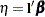. If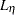and
are the confidence limits for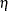on the logit scale, confidence limits for the odds or the odds ratio are obtained as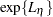and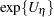.